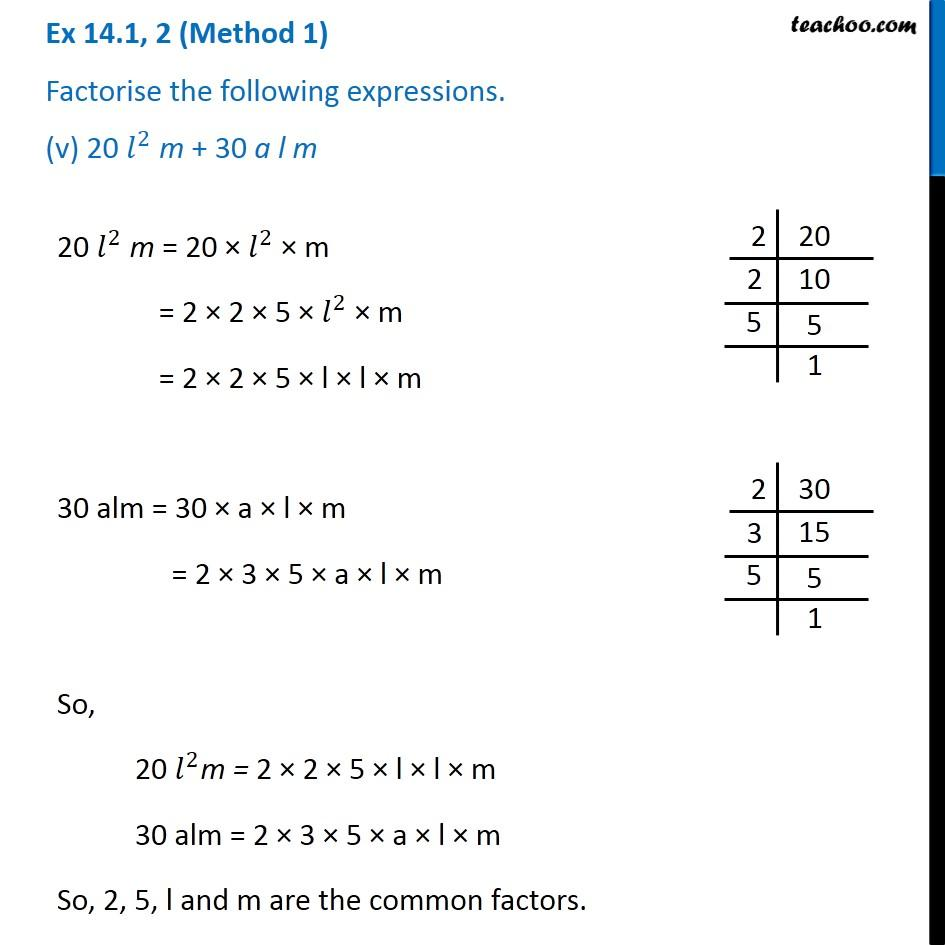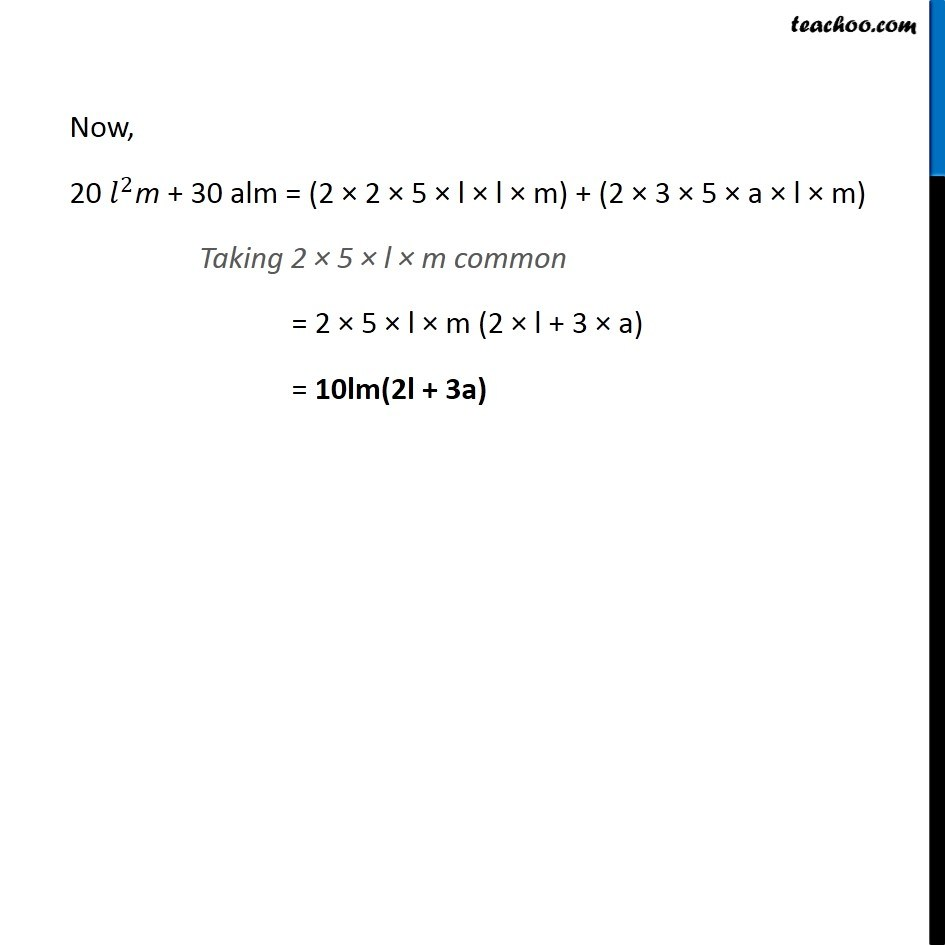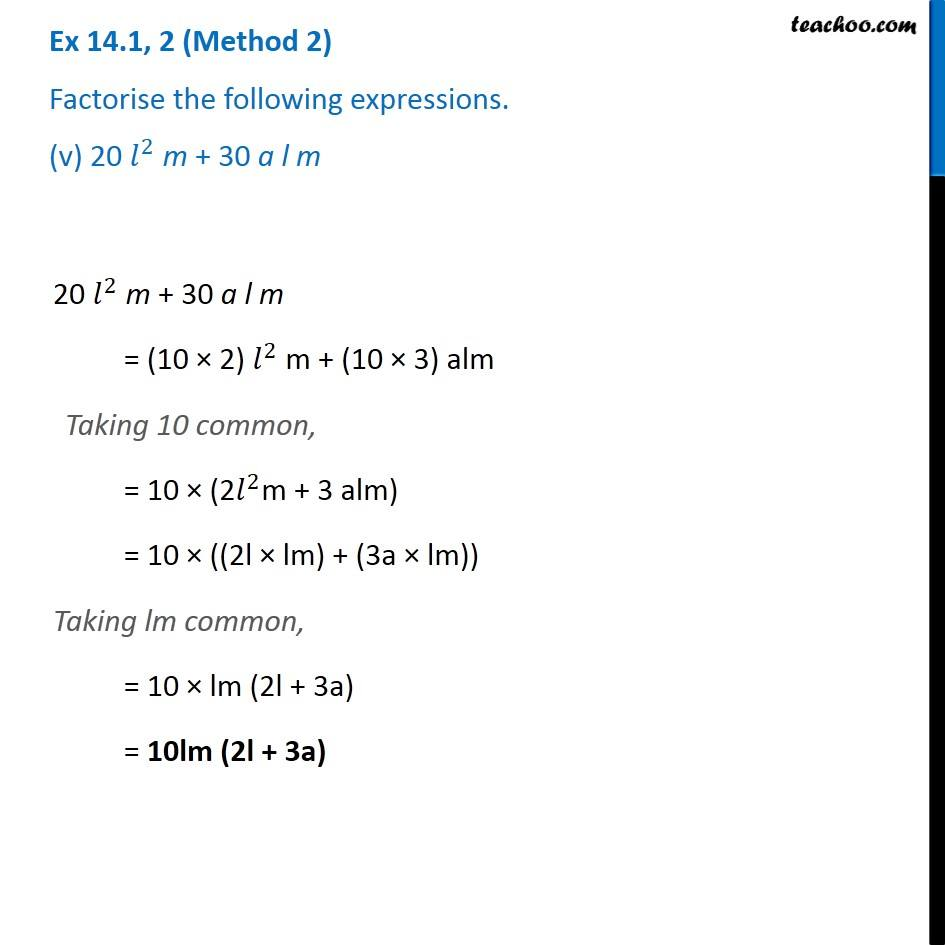Factorisation using common factors

Chapter 12 Class 8 Factorisation
Concept wiseLearn in your speed, with individual attention - Teachoo Maths 1-on-1 Class

### Transcript

Ex 12.1, 2 (Method 1) Factorise the following expressions. (v) 20 𝑙^2 m + 30 a l m 20 𝑙^2 m = 20 × 𝑙^2 × m = 2 × 2 × 5 × 𝑙^2 × m = 2 × 2 × 5 × l × l × m 30 alm = 30 × a × l × m = 2 × 3 × 5 × a × l × m So, 20 𝑙^2m = 2 × 2 × 5 × l × l × m 30 alm = 2 × 3 × 5 × a × l × m So, 2, 5, l and m are the common factors. Now, 20 𝑙^2m + 30 alm = (2 × 2 × 5 × l × l × m) + (2 × 3 × 5 × a × l × m) Taking 2 × 5 × l × m common = 2 × 5 × l × m (2 × l + 3 × a) = 10lm(2l + 3a) Ex 12.1, 2 (Method 2) Factorise the following expressions. (v) 20 𝑙^2 m + 30 a l m 20 𝑙^2 m + 30 a l m = (10 × 2) 𝑙^2 m + (10 × 3) alm Taking 10 common, = 10 × (2𝑙^2m + 3 alm) = 10 × ((2l × lm) + (3a × lm)) Taking lm common, = 10 × lm (2l + 3a) = 10lm (2l + 3a)